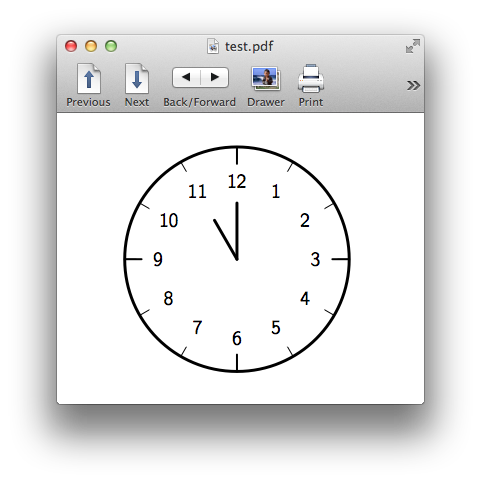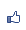Contents ...
udn網路城邦
TiKz 的foreach廻圈
2015/04/20 15:18

TiKz 提供了一個廻圈foreach，用法：

\foreach 變數[參數] in {列值} {命令}

\foreach \x in {1,2,3,0} {\x, }

1,2,...,n，意味是1, 1+1, 1+2, 1+3, ...,1+md, 如果

1+md 小於等於 n 時成立，大於 n時則不會被執行。如：

\foreach \x in {2,4,...,11} {\x, } 會是 2,4,6,8,10,

\foreach \x in {0,0.1,...,0.5} {\x, }

\foreach \x in {9,8,...,3.5} {\x, }

\foreach \x in {0.5,...,5} {\x, }

foreach的列值也可使用字母：

\foreach \x in {a,d,...,z} {\x, }

\foreach \x in {2^0,2^...,2^6} {$\x$, }

\foreach \x / \y in {1/2, a/b} {"\x \ and \y"}

\begin{tikzpicture}[line cap=round, line width=3pt]

\draw (0,0) circle (2cm);

\foreach \angle / \label in {0/3, 30/2, 60/1, 90/12, 120/11, 150/10, 180/9, 210/8, 240/7, 270/6, 300/5,330/4}

{

\draw[line width=1pt] (\angle:1.8cm)--(\angle:2cm);

\draw(\angle:1.4cm) node {\textsf{\label}};

}

\foreach \angle in {0, 90, 180, 270}

{\draw[line width=2pt] (\angle:1.7cm)--(\angle:2cm);}

\draw (0,0) --(120:0.8cm);

\draw (0,0) --(90:1cm);

\end{tikzpicture}